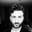Related Tags

javascript
algorithms
communitycreator

# Functional algorithms: Reverse a tree in JavaScriptdimitris papadimitriou

After lists, trees are the single most important data structure in computer science. Just about everything you do in your programming career will be related to trees. For example, JSON objects, XML, and HTML DOM are tree structures. When object-oriented JavaScript developers enter the realm of functional programming, they quickly learn how to use a map and reduce it to replace traditional for loops. Trees also have map and reduce methods.

In this shot, we are going to use a reduced method on a Binary Tree in order to reverse it in a functional way.

If we have a tree that looks like this:

Fig.1

We want to define a function, Reverse(), that will return a tree that looks like this:

Fig.2

We are going to start from an ES6 Tree structure :

class Tree {}

// node class
class Node extends Tree {
constructor(left, v, right) {
super()
this.v = v;
this.left = left;
this.right = right;
}
}
// leaf class
class Leaf extends Tree {
constructor(v) {
super()
this.v = v;
}
}

Now, we can represent the tree with objects like in Fig.1:

new Node(new Node(new Leaf(7), 3, new Leaf(3)), 6, new Node(new Leaf(8), 4, new Leaf(1)));


## Defining Pattern matching on Trees

The first thing we are going to define is a very simple matchWith method on the tree structure.

class Tree {}
class Node extends Tree {
constructor(left, v, right) {
super()
this.v = v;
this.left = left;
this.right = right;
}
matchWith( pattern) {
return  pattern.Node(this.left, this.v, this.right);
}
}
class Leaf extends Tree {
constructor(v) {
super()
this.v = v;
}
matchWith(pattern) {
return  pattern.Leaf(this.v);
}
}

This takes the pattern object as an argument:

pattern =({
Leaf: v => {}   ,
Node: (left, v, right) => { }
})


This function allows us to distinguish between Node and Leaf and is called pattern matching.

And that’s it !!! we are done. We can now define any type of recursive method on this tree. Now, we’re going to define the Reverse() function recursively.

Take a look at the following Fiddle:

class Tree {}

class Node extends Tree {
constructor(left, v, right) {
super()
this.v = v;
this.left = left;
this.right = right;
}
matchWith(pattern) {
return pattern.Node(this.left, this.v, this.right);
}
toString() {
return Node(${this.left.toString( )},${ (this.v)},${this.right.toString( )}); } } class Leaf extends Tree { constructor(v) { super() this.v = v; } matchWith(pattern) { return pattern.Leaf(this.v); } toString() { return (Leaf(${ this.v}));
}
}

Tree.prototype.reverse = function ( ) {
return this.matchWith({
Leaf: v => new Leaf(v)   ,
Node: (left, v, right) => new Node(right.reverse(),v,left.reverse())
});
}
var treeInstance  =
new Node(new Node(new Leaf(7), 3, new Leaf(3)), 6, new Node(new Leaf(8), 4, new Leaf(1)));
console.log(treeInstance.toString()) ;

var reverse  = treeInstance.reverse();
console.log(reverse.toString()) 

All the magic happens here:

Tree.prototype.reverse = function ( ) {
return this.matchWith({
Leaf: v => new Leaf(v)   ,
Node: (left, v, right) => {
return new Node(right.reverse(),v,left.reverse())
}
});
}
1. If you are on a leaf node, just return the leaf :
Leaf: v => new Leaf(v)

1. If you are on a non-leaf node, call reverse on the Left and Right Nodes**, and then return a node with those newly reversed nodes :
new Node( right.reverse(), v, left.reverse())


This method of recursive definition is called structural induction.

Let’s do another example so that you can recognize the pattern.

## Counting Nodes

Let’s say we want to count the nodes of the tree. This is how we will do it:

1. If you are on a leaf, just return $1$
2. If you are on a non-leaf node, call countNodes on the Left and Right Nodes and sum the results (also the value node counts as +1).
Tree.prototype.countNodes = function() {
return this.matchWith({
Leaf: v => 1,
Node: (left, v, right) => left.countNodes()
+ 1
+ right.countNodes()
});
}

RELATED TAGS

javascript
algorithms
communitycreator

CONTRIBUTORdimitris papadimitriou
RELATED COURSES

View all Courses

Keep Exploring

Learn in-demand tech skills in half the time